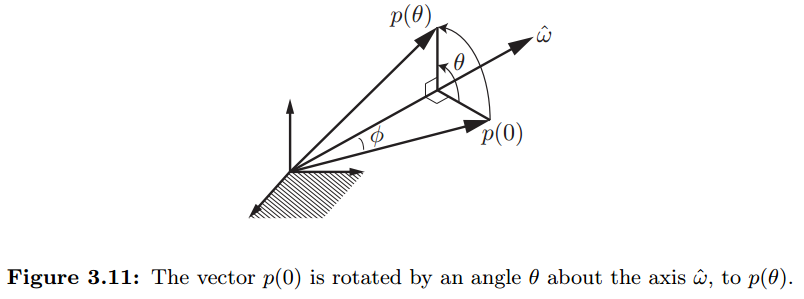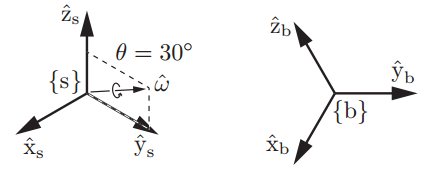### 旋转变换的指数形式

用单位向量$\stackrel{^}{\omega }$代表旋转轴，以及$\theta$代表绕该轴的旋转角度。则可以用三维向量以指数形式来描述旋转。如果将分开描述，即为Axis-Angle形式。用来描述旋转矩阵R可以有下面几种理解方法：

1. 某坐标系初始与参考坐标系{s}重合，绕轴旋转角度后当到达当前姿态，其相对于{s}的旋转矩阵为R。这种描述方法为 Axis–angle representation
2. 角速度矢量在参考坐标系{s}中描述，某坐标系初始与参考坐标系{s}重合，以角速度转动，经过单位时间到达当前姿态（可由矩阵R表示）。
3. 角速度矢量在参考坐标系{s}中描述，某坐标系初始与参考坐标系{s}重合，以角速度转动，经过时间到达当前姿态（可由矩阵R表示）。
•  Essential Results from Linear Differential Equations Theory

考虑下面的一阶线性微分方程：

矩阵指数可以根据泰勒展开式来计算：•  Exponential Coordinates of Rotations

参考下图中的描述，假设三维向量绕着转轴旋转度后到达。用代表$t$时刻向量的位置，则该过程也可以描述为：以角速度旋转（为单位角速度），从运动到$\theta$旋转时的速度可用来描述，且有：$\stackrel{˙}{p}=\stackrel{^}{\omega }×p$

用斜对称矩阵可以将向量叉乘变为矩阵与向量乘法，因此上面的微分方程可写为：$\stackrel{˙}{p}=\left[\stackrel{^}{\omega }\right]p$

若，定义斜对称矩阵由于变量可互换，则上面方程可写为：根据正弦和余弦函数的泰勒展开式：可以将上面公式进行简化。给定$\stackrel{^}{\omega }\theta \in {\mathbb{R}}^{3}$，其中为任意标量，$\stackrel{^}{\omega }\in {\mathbb{R}}^{3}$且为单位向量。则根据$\left[\stackrel{^}{\omega }\right]$进行旋转的旋转矩阵为：举个例子，下图中坐标系{b}相对于固定参考坐标系{s}的姿态可以描述为：初始时刻两坐标系一致，{b}绕单位向量$\stackrel{^}{{\omega }_{1}}=\left(0,0.866,0.5\right)$旋转后到达当前姿态。则{b}相对于{s}的旋转矩阵可以计算为：坐标系{b}的姿态可由矩阵描述，或者由单位向量$\stackrel{^}{{\omega }_{1}}=\left(0,0.866,0.5\right)$以及转角${\theta }_{1}=0.524rad$来描述，即旋转矩阵R的指数坐标为$\stackrel{^}{{\omega }_{1}}{\theta }_{1}=\left(0,0.453,0.262\right)$

在Mathematica中RotationMatrix函数可以根据转轴和转角计算旋转矩阵：•  Matrix Logarithm of Rotations

如果向量$\stackrel{^}{\omega }\theta \in {\mathbb{R}}^{3}$表达了旋转矩阵R的指数坐标，则斜对称矩阵$\left[\stackrel{^}{\omega }\theta \right]=\left[\stackrel{^}{\omega }\right]\theta$是旋转矩阵R的对数。矩阵的对数是矩阵指数的逆：或表述为斜对称矩阵形式：